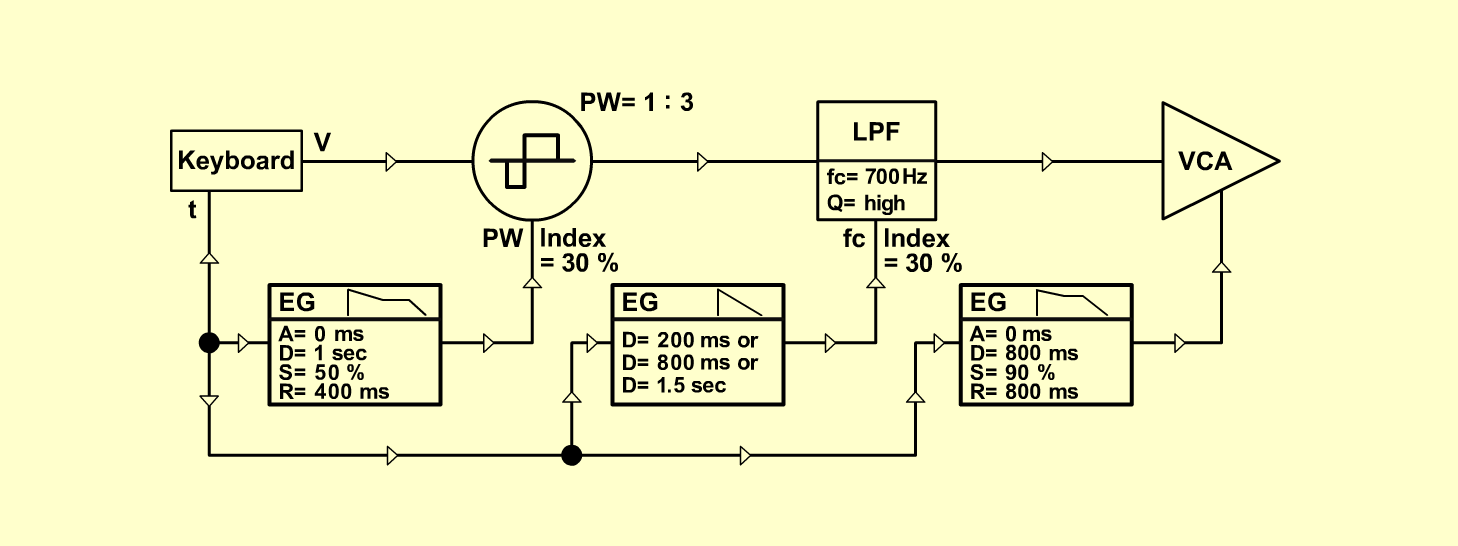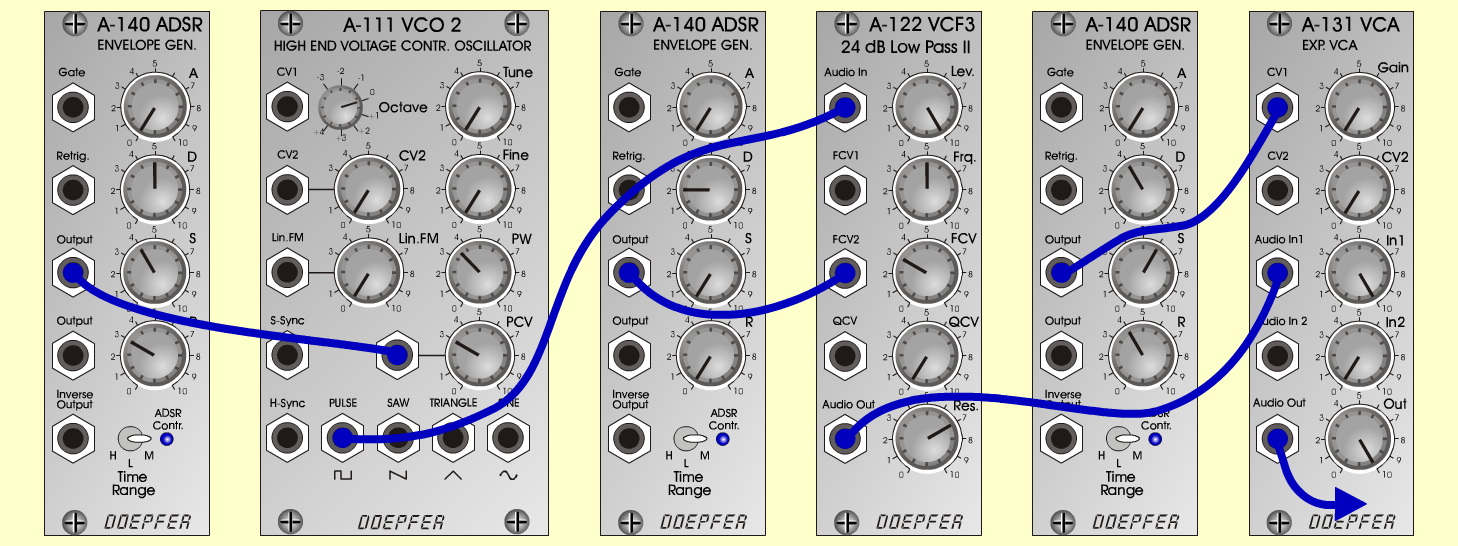Sweep-BassInstrumentsD o e p f e r A - 100 Connections: Settings: A-140/1 (Output)  <=>  A-111 (PCV) A-111 (Pulse)  <=>  A-122 (Audio In) A-140/2 (Output)  <=>  A-122 (FCV 2) A-122 (Audio Out)  <=>  A-131 (Audio In) A-140/3 (Output)  <=>  A-131 (CV 1) A-131 (Audio Out)  <=> Amplifier A-140/1 (A = 0, D = 5, S = 4, R = 3, Range = M) A-111 (PW = 3.5, PCV = 3) A-140/2 (A = 0, D = 2/4/6, S = 0, R = 0, Range = M) A-122 (Level = 10, Freq = 5, FCV  = 3, Res = 7) A-140/3 (A = 0, D = 4, S = 6, R = 4, Range = M) A-131 (Gain = 0, Audio In 1 = 10,  Audio Out = 10) Notes: A-111: The modul A-110 is also possible. Change the sweep-length with KB-Velocity.     Instead of A-140/2:      KB-Velocity  <=> A-142 (CV) or     KB-Velocity  <=> A-141 (CV D) Josef MuellerSound samples Sweep-Bass (34 Hz, Decay = 2) Sweep-Bass (65 Hz, Decay = 2) Sweep-Bass (65 Hz, Decay = 4) Sweep-Bass (65 Hz, Decay = 6) Sweep-Bass (with dig. Delay & Pan)